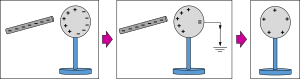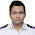## Sunday, 20 March 2016

### CBSE Class 12 - Physics - Electric Charges And Fields - Very Short Q and A

Electric Charges And Fields

### Very Short Q and ACharging By Induction credits:openclipart

Q1: Define frictional electricity.

Answer: It is the electricity developed on bodies when they rubbed against each other.

Q2: What is the smallest amount of charge?

Answer: Charge on an electron or proton is ± 1.62 × 10-19 C

Q3: State the two basic properties of electric charge?

Answer:  i. Electric charge is quantised.
ii. Electric charge is always conserved.

Q4: Define Dielectric constant.

Answer: The Dielectric constant of a medium is the ratio of the force between two charges placed at a certain distance apart in vacuum(or air) to the force between the same two charges at the same distance apart in that medium.

Q5: What is induction?

Answer: It is a process of charging a body without making physical contact.

Q6 (CBSE): Why does the ebonite rod gets negative charged on rubbing with fur?

Answer: On rubbing, fur loses electrons and ebonite gains electrons. Electrons in fur are less tightly bound than electrons in ebonite.

Q7: Define electric field.

Answer: It is the space around an electric charge where its effect can be experienced.

Q8: Why do two electric field lines never cross each other at any point?

Answer: The force by electric field line give direction to the electric field. When two lines of electric field intersect, there will be resultant force and single line of direction. At the point of intersection there can't be two directions of the electric field.

Q9: What is the SI unit of electric dipole moment?

Answer: Coulomb Metre (Cm)

Q10: What is Guassian Surface?

Answer: Any closed imaginary surface around the charge distribution where Guassian theorem can be applied to find electric field, the surface is called Guassian Surface.

Q11: What is the strength of the electric field inside a charged spherical shell?1.